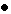II. MEASUREMENT METHODS COMPARISON When making measurements between a pair of frequency standards or clocks, it is desirable to have less noise in the measurement system than the composite noise in the pair of standards being measured. This places stringent requirements on measurement systems as the state-of-the-art of precision frequency and time standards has advanced. As will be shown, perhaps one of the greatest areas of disparity between measurement system noise and the noise in precision standards is in the area of time difference measurements. Commercial equipment can measure time differences to about 10-11 s, but the time fluctuations second to second of state-of-the-art standards is as good as 10-13 s. The disparity is unfortunate because if time differences between two standards could be measured with adequate precision then one may also know the time fluctuations, the frequency differences, and the frequency fluctuations. In fact, one can set up an interesting hierarchy of kinds of measurement systems: 1) those that can measure time, x(t); 2) those that can measure changes in time or time fluctuations d x(t); 3) those that can measure frequency, n (y / (n -n 0)/n 0); and 4) those that can measure changes in frequency or frequency fluctuations, d n (d y / d n /n 0). As depicted in table 2.1, if a measurement system is of status 1 in this hierarchy, i.e., it can measure time, then time fluctuations, frequency and frequency fluctuations can also be deduced. However, if a measurement system is only capable of measuring time fluctuations (status 2 - table 2.1), then time cannot be deduced, but frequency and frequency fluctuations can. If frequency is being measured (status 3 - table 2.1), then neither time nor time fluctuations may be deduced with fidelity because essentially all commercial frequency measuring devices have "dead time" (technology is at a point where that is changing with fast data processing speeds that are now available). Dead time in a frequency measurement destroys the opportunity of integrating the fractional frequency to get to "true" time fluctuations. Of course, if frequency can be measured, then trivially one may deduce the frequency fluctuations. Finally, if a system can only measure frequency fluctuations (status 4 - table 2.1), then neither time, nor time fluctuations, nor frequency can be deduced from the data. If the frequency stability is the primary concern then one may be perfectly happy to employ such a measurement system, and similarly for other statuses in this measurement hierarchy. Obviously, if a measurement method of status 1 could be employed with state-of-the-art precision, this would provide the greatest flexibility in data processing. From section 1, the dual mixer time difference system is such a method. Main PageTable of ContentsGo to section: Summary and IntroductionIIIIIIIVVVIVIIVIIIIXXXIXIIConclusionReferences